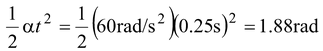# Rotational Kinematics

## Rotational Motion of Class 11

Consider a body of arbitrary shape rotating about a fixed axis ‘O’ as shown in figure(10.2). In a given interval all the particles lying on the line OA move to their corresponding positions lying on OB.

 Although the particles of the body have different linear displacements, they all have the same angular displacement θ, which is given by θ = s/r   (10.1) The average angular velocity of the body for a finite time interval is given by ωav =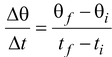(10.2) The unit of angular velocity is radian per second (rad/s). The instantaneous angular velocity is defined as ω =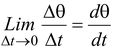(10.3)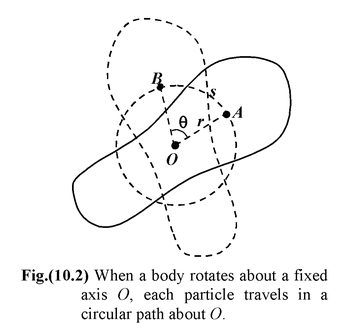It is the rate of change of the angular position θ with respect to time. It is a vector quantity. The direction of angular velocity is given by the right-hand rule. We hold the right hand such that when the fingers of the right hand curve in the sense of rotation, the thumb points in the direction of ω.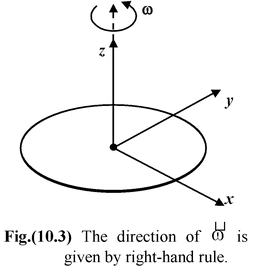### Period and Frequency of Revolution

The period T is the time for one revolution and the frequency f is the number of revolutions per second (rev/s). The period and frequency are related as

f = 1/T   (10.4)

If the angular velocity is constant, the instantaneous and average velocities are equal.

In terms period T and frequency f the angular velocity is given by

ω = 2π/T = 2πf  (10.5)

The relation between linear speed and angular speed is obtained by differentiating
equation (10.1) with respect to time.

dθ/dt = 1/r ds/dt or ω = v/r

orv = ωr (10.6)

Although all particles have the same angular velocity, their speeds increase linearly with distance from the axis of rotation.

The average angular acceleration is defined as

αav =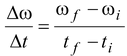(10.7)

and the instantaneous angular acceleration is defined as

α =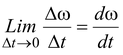(10.8)

Angular acceleration is a vector quantity measured in rad/s2.

The Constant Angular Acceleration Model

When the angular acceleration is constant, we can find the change in angular velocity by integrating equation (10.8)

dω = α dt

or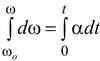or ω - ωo = αt

or(10.9)

Substituting the value of ω in equation (10.3), we get

dθ = ωdt = (ωo + αt)dt

On integrating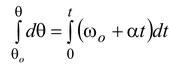θ - θo = ωot + 1/2at2

or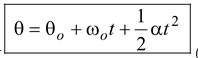(10.10)

On eliminating t from equation (10.9) and (10.10), we get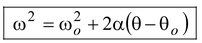(10.11)

The equations (10.9), (10.10) and (10.11) are called the equations of rotation kinematics for constant angular acceleration.

A particle moving in a circular path with speed v has a centripetal (or radial) acceleration

 ar =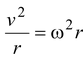(10.12) If there is angular acceleration, the speed of the particle changes and thus we can find the tangential acceleration at =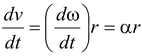(10.13) The net acceleration is: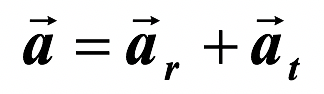The magnitude of acceleration is given by a =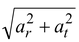(10.14)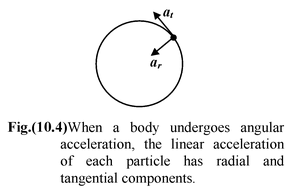Table 10.1 Analogy Between Rotational Kinematics and Linear Kinematics

 Quantity Linear Rotational 1.Position x θ 2.Displacement Δx Δθ 3.Velocity v = dx/dt ω = dθ/dt 4.Acceleration a = dv/dt α = dω/dt v = ∫a dt + c ω = ∫αdt + c x = ∫vdt' + c' θ = ∫ωdt + c' 5. Equations of Kinematics v = vo + at ω = ωο + αt x = xo + vot + 1/2at2 θ = θο + ωοt + 1/2αt2 v2 − vo2 = 2a(x- xo) ω2 − ωο2 = 2α(θ − θο)

Example 10.1

A disc starts rotating with constant angular acceleration of π rad/s2 about a fixed axis perpendicular to its plane and through its centre.

(a)Find the angular velocity of the disc after 4 s.

(b)Find the angular displacement of the disc after 4 s and

(c)Find number of turns accomplished by the disc in 4 s.

Solution

ω0 = 0

t = 4 sec

(a)ω = 0 + (π rad/s2) × 4 sec = 4π rad/s.

(b)θ = 0 + (π rad/s2) × (16 s2) = 8 π rad.

(c)Let the number of turns be n

Example 10.2

A wheel rotates with an angular acceleration given by α = 4at3 - 3bt2 , where t is the time and a and b are constants. If the wheel has initial angular speed ω0, write the equations for the:

(i) angular speed

(ii) angular displacement.

Solution

(i)From equation (10.8), we know

dω = α dt

Integrating both sides, we get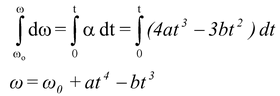(ii)From equation (10.3), we know

dθ = ωdt

On integrating both the sides, we get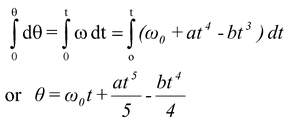Example 10.3

A flywheel of radius 20 cm starts from rest, and has a constant angular acceleration of 60 rad/s2. Find

(a)the magnitude of the net linear acceleration of a point on the rim after 0.15 s

(b) the number of revolutions completed in 0.25 s

Solution

(a)The tangential acceleration is constant and given by

at = αr = (60 rad/s2)(0.2 m) = 12 m/s2

In order to calculate the radial acceleration we first need to find the angular velocity at the given time. From equation (10.9), we have

ω = ωo + αt = 0 + (60 rad/s2)(0.15 s) = 9 rad/s

Then using equation (10.12), we have

ar = ω2r = (81 rad2/s2)(0.2 m) = 16.2 m/s2

The magnitude of the net linear acceleration is

a == 20.2 m/s2

(b)From equation (10.10),

0 =